## Differentiation Homework

### d2vid

Posted 03 October 2006 - 03:27 PM

Hello there,

I'm stuck on question 6. Could anyone explain it to me? In general I know what is it about, I just don't know how to get values of this letters.

I attach the question in PDF.

### John

Posted 03 October 2006 - 03:37 PM

sketch the graph of the derivitive and they should become clear### d2vid

Posted 03 October 2006 - 03:47 PM

Do you mean to make a graph and get the values from graph? There isn't any way to do it without sketching (I mean get the values from calculating)?

### John

Posted 03 October 2006 - 05:29 PM

You will not get a number, as you do not have the equation, or enough information to calculate the values.

But if you sketch the graph of the derivitive(if you dont know how to this just ask and i will explain) and you should be able to figure it out.

EDIT: I wasnt thinking properly lol

### d2vid

Posted 03 October 2006 - 05:48 PM

Hmm, ok.. I just thought that if I have the angle ( tan 0 (theta) = m ), then I have the gradient, so I can get some values without drawing. Derivative is like getting graph of f'(x) from f(x), if I'm wrong, correct me### Steve

Posted 03 October 2006 - 05:58 PM

No, you're quite right. You can find a value for each of those asked in part (a).

Here's a hint: The gradient of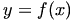at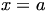is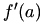.

Notice that (b,c) and (d,e) are stationary points, what is the gradient at a stationary point?

Once you see the relationship between the derivative and the gradient, you can also find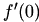.

Any thoughts?

### d2vid

Posted 03 October 2006 - 06:12 PM

No, you're quite right. You can find a value for each of those asked in part (a).

Here's a hint: The gradient ofatis.

Notice that (b,c) and (d,e) are stationary points, what is the gradient at a stationary point?

Once you see the relationship between the derivative and the gradient, you can also find.

Any thoughts?

I knew there must some way to work it out. Gradient at stationary points is always 0.

So now I know that gradient at this points equals to 0, so, is it? But how to work out the number?

### Steve

Posted 04 October 2006 - 12:51 PM

Yes, you're right about, but (d,e) is also a stationary point so you can apply the same argument for.

You can work out the gradient of the tangent, and this is a tangent at x = 0. Since the gradient of the tangent at x = a is given by, this means thatis equal to whatever the gradient of the tangent is at x = a.

I'm deliberately not just telling you the answer, does that help?

### John

Posted 04 October 2006 - 12:53 PM

Yes, you're right about, but (d,e) is also a stationary point so you can apply the same argument for.

You can work out the gradient of the tangent, and this is a tangent at x = 0. Since the gradient of the tangent at x = a is given by, this means thatis equal to whatever the gradient of the tangent is at x = a.

I'm deliberately not just telling you the answer, does that help?

I dont know why i didnt actually say that, becuase i wrote it down >_<

Maybe i should stop doing maths while hungry lol

### d2vid

Posted 04 October 2006 - 01:54 PM

Yes, you're right about, but (d,e) is also a stationary point so you can apply the same argument for.

You can work out the gradient of the tangent, and this is a tangent at x = 0. Since the gradient of the tangent at x = a is given by, this means thatis equal to whatever the gradient of the tangent is at x = a.

I'm deliberately not just telling you the answer, does that help?

I know you won't give me an answerBut I now understand, thanks for help you allAt a turning point, the tangent to the curve has gradient zero i.e.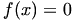Thus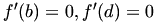The tangent athas gradient tan(45°) i.e. 1 so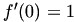Can you check and correct my work if i'm wrong?

### d2vid

Posted 04 October 2006 - 03:08 PM

Oh, I have another problem.. I am not quite sure about the answer to question 1f

I got two answers, one is 5X to the power of 4, and the second one is 3X squared + 2ab X to the power of -3.

I am not sure what to do with X to the power of 3 - ab/X squared.

I hope I described it well.### Steve

Posted 04 October 2006 - 05:39 PM

ThusThe tangent athas gradient tan(45°) i.e. 1 soCan you check and correct my work if i'm wrong?

Perfect.

For 1(f), you just have to remember that a and b are constants, so you just treat them like you would any number. You can write: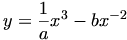Then differentiate as normal, treating a and b as constants, i.e. just use the rule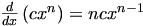on each term.

(I got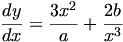.)

### d2vid

Posted 04 October 2006 - 05:55 PM

Thank you guys! Much appreciated! I will be helping people too when I'll finish the courseThanks again!

### Steve

Posted 04 October 2006 - 06:08 PM

No problem, that's why we're here!